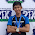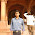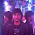## Electron Devices Objective Type Questions

1.When a piece of copper and another of germanium are cooled from room temperature to 800 K then the resistance of -
a) Each of them increases
b) Each of them decreases
c) Copper increases and germanium decreases
d) Copper decreases and germanium increases

2.When a sample of germanium and silicon having same impurity density are kept at room temperature then?
a) Both will have equal value of resistivity
b) Both will have equal value negative resistivity
c) Resistivity of germanium will be higher than that of silicon
d) Resistivity of silicon will be higher than that of germanium

3.When an RC driving point impedance function has zeros at s= -2 and s=-5 then the admissible poles for the function would be?
a) s = 0; s = -6
b) s = 0; s = -3
c) s = 0; s = -1
d) s = -3; s = -4

4.A transistor has CE parameter as h
ie = 10kW, hre=20 x 10-4 , hse = 100, hoe = 25 ms. The hib for this transistor will be-
a) 100 W
b) 99.01 W
c) 5m W
d) 101kW

5.Which one of the following conditions for Z parameters would hold for a two port network containing linear bilateral passive circuit elements?
a) Z11 = Z22
b) Z12Z21 = Z11Z22
c) Z11Z12 = Z22Z21
d) Z12 = Z21

6.A transistor is in active region when-
a) IB =  βIC
b) IC= βIB
c) IC=IE
d) IC=IB

7.In a JFET gates are always?
a) Forward biased
b) Reverse biased
c) Unbiased
d) None

8.In schotty barrier diode current flows because of?
a) Majority carriers
b) Minority carriers
c) Majority and minority carriers
d) None

9.The early voltage of a BJT is VA = 75V. The minimum required collector current, such that the output resistance is at least r0 = 200kΩ, is
(a) 1.67mA
(b) 5mA
(c) 0.375mA
(d) 0.75mA

10.TVS diode is used for
(a) Voltage protection
(b) Current protection
(c) ESD protection
(d) None of the above

 The amplifier is which current is proportional to the signal voltage, independent of source load resistance is called
a) Current Amplifier
b) Voltage Amplifier
c) Transresistance amplifier
d) Transconductance amplifier

 Alternatively the characteristic impedance is called

a) Surge Impedance
b) Match Impedance
c) Alternative Impedance
d) Reflected Impedance

 In an RL circuit after a very long time of application of step voltage the inductance L is represented in its equivalent circuit as Incorrect!
a) Open Circuit
b) Short Circuit
c) L / 2
d) 2L

 By passing a triangular wave through a differentiating circuit the output wave shape is
a) Spikes
b) Squarewave
c) Sawtooth
d) Sinewave

 Clipper circuits are used to obtain any one of the following waveforms
a) Sharper
b) Rectified
c) Fast Rising
d) Smaller Amplitude

Solution Hint:
 When the temperature increases, the resistance of conductor(copper) decreases and resistance of semiconductor(germanium) increases
 In the FET, the gate terminal is electrically isolated from the channel. ie, it is always unbiased.
 TVS stands for Transient Voltage suppressor. It is used for transient voltage protection.
 The clipper circuit will clip part of the waveform. so it can be used for smaller amplitude of the waveform.
6:00 AM

1.very useful question plz provide formula as well and plz mail some electrical eng related objective typ qstn on email. id shailendergpt5@gmail.com

2.Vvvv awsm. But plz provide wit brief explanation. Send questions to muttaraja009@gmail.com

3.Vvvv awsm. But plz provide wit brief explanation. Send questions to muttaraja009@gmail.com

4.Can anyone explain the answer of question no 3

5.I think the answer in question 14 is incorrect since the derivative of triangle wave is a square wave, so it should be (B).

6.I think answer of question no 14 will be
B> Squarewave

7.question number 14 answer is B please check and update the answer

8.IN QUESTION 8. SCHOTTKY DIODE, THE CURRENT FLOW IS BY MAJORITY CARRIERS NOT MINORITY CARRIERS. HENCE IT IS FASTEST THAN OTHER TYPES IN RECOVERY TIME.